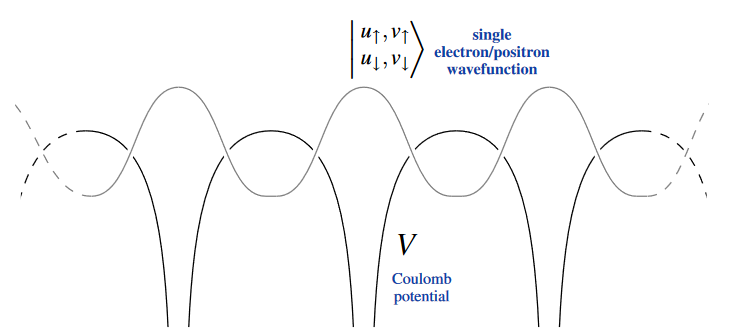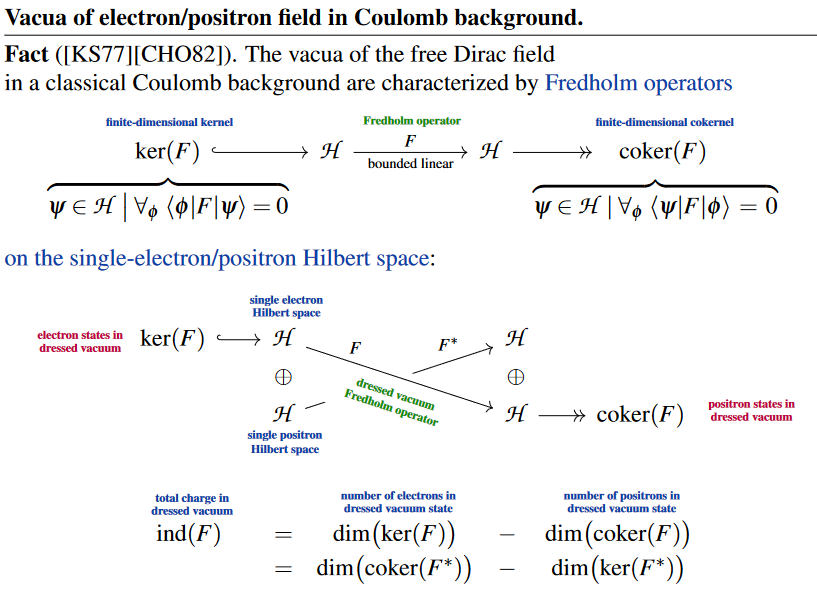# nLab Dirac field

Contents

### Context

#### Fields and quanta

fields and particles in particle physics

and in the standard model of particle physics:

force field gauge bosons

scalar bosons

flavors of fundamental fermions in the
standard model of particle physics:
generation of fermions1st generation2nd generation3d generation
quarks ($q$)
up-typeup quark ($u$)charm quark ($c$)top quark ($t$)
down-typedown quark ($d$)strange quark ($s$)bottom quark ($b$)
leptons
chargedelectronmuontauon
neutralelectron neutrinomuon neutrinotau neutrino
bound states:
mesonslight mesons:
pion ($u d$)
ρ-meson ($u d$)
ω-meson ($u d$)
f1-meson
a1-meson
strange-mesons:
ϕ-meson ($s \bar s$),
kaon, K*-meson ($u s$, $d s$)
eta-meson ($u u + d d + s s$)

charmed heavy mesons:
D-meson ($u c$, $d c$, $s c$)
J/ψ-meson ($c \bar c$)
bottom heavy mesons:
B-meson ($q b$)
ϒ-meson ($b \bar b$)
baryonsnucleons:
proton $(u u d)$
neutron $(u d d)$

(also: antiparticles)

effective particles

hadrons (bound states of the above quarks)

solitons

in grand unified theory

minimally extended supersymmetric standard model

superpartners

bosinos:

dark matter candidates

Exotica

auxiliary fields

## Spin geometry

spin geometry

Dynkin labelsp. orth. groupspin grouppin groupsemi-spin group
SO(2)Spin(2)Pin(2)
B1SO(3)Spin(3)Pin(3)
D2SO(4)Spin(4)Pin(4)
B2SO(5)Spin(5)Pin(5)
D3SO(6)Spin(6)
B3SO(7)Spin(7)
D4SO(8)Spin(8)SO(8)
B4SO(9)Spin(9)
D5SO(10)Spin(10)
B5SO(11)Spin(11)
D6SO(12)Spin(12)
$\vdots$$\vdots$
D8SO(16)Spin(16)SemiSpin(16)
$\vdots$$\vdots$
D16SO(32)Spin(32)SemiSpin(32)

string geometry

# Contents

## Idea

In physics, specifically in field theory, a Dirac field is an electromagentially charged and possibly massive a fermionic spinor field.

This includes fermions in the standard model of particle physics, such as electrons, quarks, neutrinos and muons, after the Higgs mechanism has equipped them with mass.

Mathematically, the Dirac field is the field whose field bundle is a spinor bundle with typical fiber a Dirac representation in odd super-degree and whose equation of motion is a Dirac equation.

Notice that there are other spinor fields which would not be called “Dirac fields”, such as those transforming in a Majorana representation or a Weyl representation, or for example the gravitino field which would be called a Rarita-Schwinger field.

## Properties

### Free Dirac field in Couloumb background

In solid state physics, the electrons described by the Dirac equation are encountered in the electromagnetic background field that is sourced by atomic nuclei such as those on the lattice sites of a crystal.

In a common approximation used in condensed matter theory for computing ground state-properties of crystals, the nuclei are typically treated as motionless (due to their large mass compared to that of the electrons), hence sourcing a static Coulomb background field, while the electromagnetic interaction of the electrons with each other is neglected. In this approximation one is dealing with a “free Dirac field in a static Coulomb background”.(graphics from SS22, cf. Rößler 2004, Fig. 2.1)

The vacua/ground state of the free Dirac field in such a “classical Coulomb background” (review in Thaller 92, Sec. 10) have been computed in Klaus & Scharf 77 and found to be characterized by Fredholm operators (amplified in Carey, Hurst & O’Brien 82) whose kernel/cokernel are the Hilbert spaces of single electron/positron quantum states which are occupied in the charged vacuum, hence whose Fredholm index is the total charge in this vacuum.(graphics from SS 22, Sc 22)

## Reference

### General

A concise collection of the Dirac field over Minkowski spacetime is in

with background on spinor fields in

A textbook account of the interacting Dirac field in the context of causal perturbation theory:

Textbook account of the free Dirac field but possiblyin an electromagnetic background field:

### Free Dirac field in Coulomb background

Discussion of the charged vacua/ground states of the free Dirac field in a strong Coulomb background field (such as that sourced by atomic nuclei on a crystal lattice):

Identification of the vacua/ground states of the free Dirac field in a Coulomb background field:

and the observation that these vacua are characterized by Fredholm operators whose index is the total charge in the vacuum:

This curious observation seems not to have been followed up on much, but some related references are listed in Thaller 92, p. 319.

Last revised on September 7, 2023 at 06:59:04. See the history of this page for a list of all contributions to it.